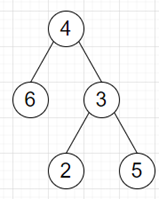# Program to find sum of all numbers formed by path of a binary tree in python

Suppose we have a binary tree where each node is containing a single digit from 0 to 9. Now each path from the root to the leaf represents a number with its digits in order. We have to find the sum of numbers represented by all paths in the tree.

So, if the input is likethen the output will be 680 as 46 (4 → 6), 432 (4 → 3 → 2), 435 (4 → 3 → 5), and their sum is 913.

To solve this, we will follow these steps −

• Define a function solve() . This will take root, string:= blank string
• if root and not root.left and not root.right is non-zero, then
• return int(string + str(value of root))
• total := 0
• if left of root is not null, then
• total := total + numeric value of solve(left of root, string concatenate value of root)
• if right of root is not null, then
• total := total + numeric value of solve(right of root, string concatenate value of root)

Let us see the following implementation to get better understanding −

## Example

Live Demo

class TreeNode:
def __init__(self, data, left = None, right = None):
self.val = data
self.left = left
self.right = right

class Solution:
def solve(self, root, string=""):
if root and not root.left and not root.right:
return int(string + str(root.val))

total = 0
if root.left:
total += int(self.solve(root.left, string + str(root.val)))

if root.right:
total += int(self.solve(root.right, string + str(root.val)))

ob = Solution()
root = TreeNode(4)
root.left = TreeNode(6)
root.right = TreeNode(3)
root.right.left = TreeNode(2)
root.right.right = TreeNode(5)
print(ob.solve(root))

## Input

root = TreeNode(4)
root.left = TreeNode(6)
root.right = TreeNode(3)
root.right.left = TreeNode(2)
root.right.right = TreeNode(5)

## Output

913